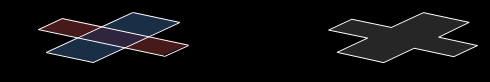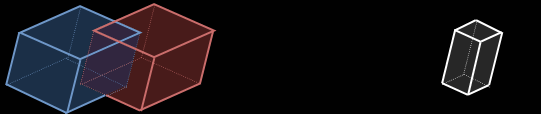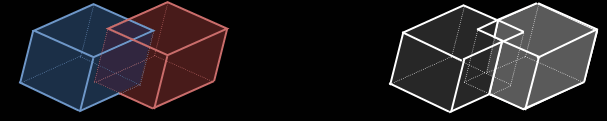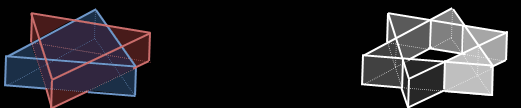top of page# Geometric Boolean Operators

The Geometric Boolean Operation Algorithm Suite contains four primary and one specialized Boolean operations that can be applied to surface and volumetric objects:

1. Union

2. Intersect

3. Difference

4. Subtract

5. Subtract and Keep

Union

A geometric Boolean union is a merging operation that combines 2 or more objects into a single object. The end result is a composit surface or volume with any overlapping internal features removed. The figure below shows the union of two cubes.The red and blue cubes are of equal heights and are aligned so that the top and bottom faces of both are coplanar. The Union algorithm intersects all of the faces of the two cubes and determines which faces are inside the volume of other cube and removes those from the composite face list. The fragmented coplanar faces are then merged to create a minimal volumetric object that spans the same spatial area as the individual pair.

The same process is used for planar objects. The figure below shows the union of two coplanar rectangles.The geometric Boolean algorithm can also union several objects at once to create a composite volume or surface. The figure below shows the algorithm results for a 5 object union.If the 5 objects in the left are all selected at once for a union operation, the algorithm searches for overlapping volumes between each object and determines that the red, green, and blue volumes overlap and the orange and cyan cubes overlap. The algorithm then only unions the overlapping volumes to create the two gray objects shown on the right.

Intersect

Boolean intersection merges two objects and only returns the overlapping regions. All regions on one object that are not located inside the other are removed. The example below shows the intersection result from the same two cube example shown for the union.Unlike the union operation, depending on the geometry of the object several objects may be returned from an intersection. The example below shows such a case. The blue E-shaped volume is intersected with the red extruded rectangle. The resulting objects are the three gray cubes on the right.Difference

Boolean difference is the opposite operation as the union. In a Boolean difference only the non-overlapping regions are kept and the overlapping regions are removed. Again, using the cube example, the result of a difference is shown below.Like the intersection, the difference can result in several output objects. This is shown in the two triangle example below. The intersection of the red and blue extruded triangles results in the 6 output objects shown on the right.Subtract

Boolean subtraction is the only one of the Boolean operations that is non-commutative. Change the order of the two objects changes the result. In the two cube example, if the red is subtracted from the blue, the red object is removed completely and the region that had overlapped between the two has been removed from the blue cube. The opposite is true if the blue is subtracted from the blue.As with the intersection method, subtract can return multiple objects. In the example below the extruded rectangle is subtracted from the E-shaped volume, returning the four gray volumes on the right.Subtract and Keep

Boolean subtraction is the only one of the Boolean operations that is non-commutative. Change the order of the two objects changes the result. In the two cube example, if the red is subtracted from the blue, the red object is removed completely and the region that had overlapped between the two has been removed from the blue cube. The opposite is true if the blue is subtracted from the blue.As with the intersection method, subtract can return multiple objects. In the example below the extruded rectangle is subtracted from the E-shaped volume, returning the four gray volumes on the right.bottom of page